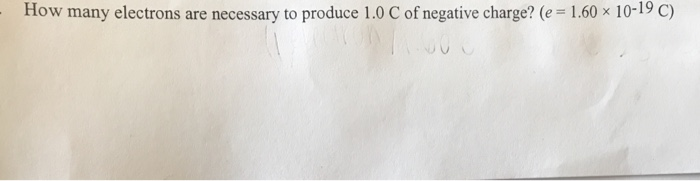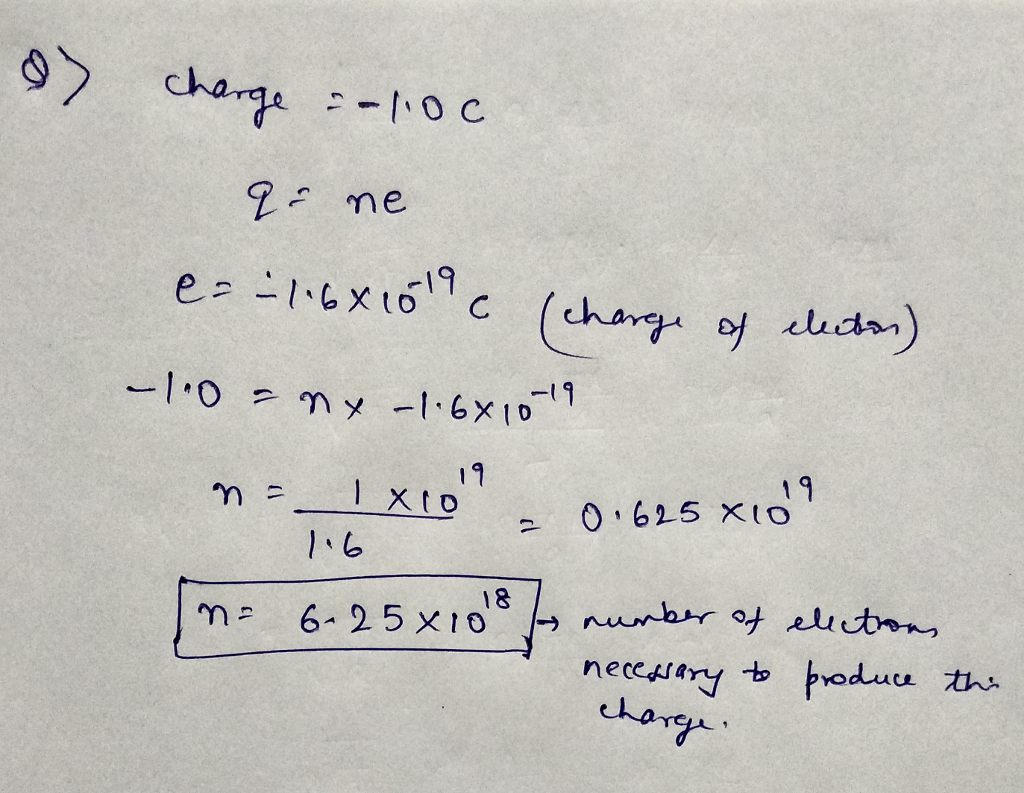# How many electrons are necessary to produce 1.0 c of negative charge? (e = 1.60 × 10-19 c)

How
many electrons are necessary to produce 1.0 C of negative charge?
(e=1.60×10^-19C)How many electrons are necessary to produce 1.0 C of negative charge? (e 1.60 x 10-19 C)

Please like. Thank You
a) charge = -1.0 c q = ne e = -1.6 times 10^- 19 c (charge of electron) – 1.0 = n times – 1.6 times 10^- 19 n = 1 times – 1.6 times 10^- 19 n = 1 times 10^19/1.6 = 0.625 times 10^19 n = 6.25 times 10^18 rightarrow number of electron necessary to produce This charge.